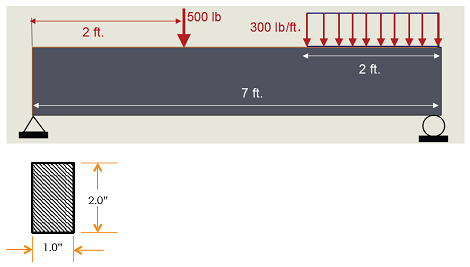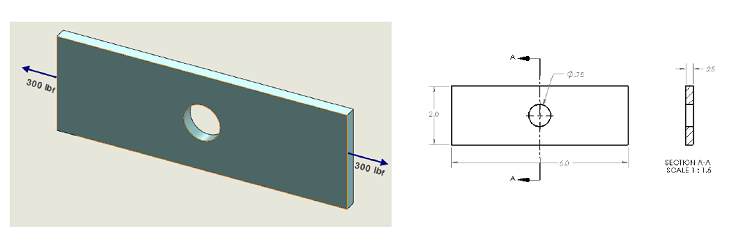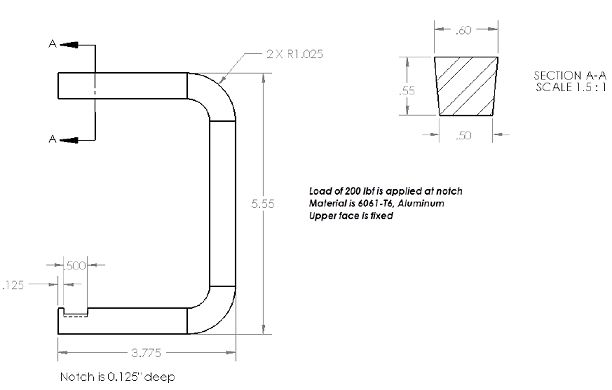### Determine the analytical solution for the maximum stress

Assignment Help Mechanical Engineering
##### Reference no: EM131570

Assignment 1

Problem 1:

Determine the analytical solution for the maximum stress of the beam shown; compare with stress results from SolidWorks. Draw shear and moment diagrams and print them from the beam diagram menu in the SolidWorks Simulation study. The beam should be assigned an Alloy Steel material.Problem 2:

The following machine component is loaded as shown. It is fabricated from Al 6061-T6.Determine the analytical solution to this problem and compare results for a model consisting of ½ the plate and ¼ of the plate. Submit screen shots of the boundary conditions and loadings for both models along with normal stress plots. Calculate the percent error between the analytical solution and the solution obtained with each model.Problem 3:

Determine the analytical solution for the maximum stress in the member shown. Submit screen shots that show the shape of the elements that result with a global element size of 0.15 a tolerance of 0.0075. Capture a copy of the mesh details and include it with the meshed model. Turn in a plot of the von Mises stresses in psi. Calculate the % error between the analytical and numerical stress values.### Write a Review